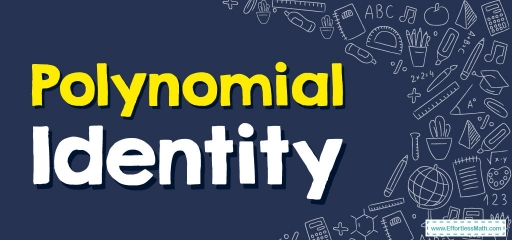# Polynomial Identity

Polynomial identity is a mathematical fact that helps us to quickly solve expressions that contain large numbers and powers. In this guide, you will learn more about polynomial identity.Polynomial identities are equations that hold true for all possible values of the variable. When solving problems with polynomial identities, identify the pattern to see if the form is simplified or factored form, and then apply the identity and solve.

## A step-by-step guide topolynomial identity

Polynomial identity refers to an equation that is always true regardless of the values assigned to the variables. We use polynomial identities to expand or factorize polynomials.

We must learn polynomial identities in mathematics. Four important identities of the polynomial are listed below.

• $$\color{blue}{\left(a\:+\:b\right)^2=\:a^2+\:2ab\:+\:b^2}$$
• $$\color{blue}{\left(a\:−\:b\right)^2=\:a^2−\:2ab\:+\:b^2}$$
• $$\color{blue}{\left(a\:+\:b\right)\left(a\:−\:b\right)\:=\:a^2−\:b^2}$$
• $$\color{blue}{\left(x\:+\:a\right)\left(x\:+\:b\right)\:=\:x^2+\:x\left(a\:+\:b\right)\:+\:ab}$$

Apart from the simple polynomial identities mentioned above, there are other identities of polynomials. Here are some of the most common polynomial identities used:

• $$\color{blue}{\left(a\:+\:b\:+\:c\right)^2=\:a^2+\:b^2+\:c^2+\:2ab\:+\:2bc\:+\:2ca}$$
• $$\color{blue}{\left(a\:+\:b\right)^3=\:a^3+\:3a^2b\:+\:3ab^2+\:b^3}$$
• $$\color{blue}{\left(a\:−\:b\right)^3=\:a^3−\:3a^2b+\:3ab^2−\:b^3}$$
• $$\color{blue}{\left(a\right)^3+\:\left(b\right)^3=\:\left(a\:+\:b\right)\left(a^2−\:ab\:+\:b^2\right)}$$
• $$\color{blue}{\left(a\right)^3−\:\left(b\right)^3=\:\left(a\:−\:b\right)\left(a^2+\:ab\:+\:b^2\right)}$$
• $$\color{blue}{\left(a\right)^3+\:\left(b\right)^3+\:\left(c\right)^3−\:3abc\:=\:\left(a\:+\:b\:+\:c\right)\left(a^2+\:b^2+\:c^2−\:ab\:−\:bc−ca\right)}$$

### Polynomial Identity – Example 1:

Using polynomial identities, find $$\left(3x\:-2y\right)^2$$.

Solution:

To solve polynomial, use this identity: $$\left(a\:−\:b\right)^2=\:a^2−\:2ab\:+\:b^2$$

Here, $$a=3x$$ and $$b=2y$$.

Then: $$\left(3x\:−\:2y\right)^2=\:\left(3x\right)^2−\:2\left(3x\right)\left(2y\right)+\left(2y\right)^2=\:9x^2−\:12xy\:+\:4y^2$$

Therefore, $$\left(3x\:−\:2y\right)^2=\:9x^2−\:12xy\:+\:4y^2$$

## Exercises for Polynomial Identity

### Simplify each expression.

1. $$\color{blue}{\left(6x\:+\:5y\right)^2\:+\:\left(6x\:-\:5y\right)^2}$$
2. $$\color{blue}{\left(4x^3-3\right)^2}$$
3. $$\color{blue}{\left(2x^2+y^3\right)^2\left(3x^2+y^3\right)}$$
4. $$\color{blue}{\left(5x-2y\right)^3}$$
1. $$\color{blue}{72x^2+50y^2}$$
2. $$\color{blue}{16x^6-24x^3+9}$$
3. $$\color{blue}{12x^6+16x^4y^3+4x^2y^6+3y^6x^2+y^9}$$
4. $$\color{blue}{\:125x^3-150x^2y+60xy^2-8y^3}$$

### What people say about "Polynomial Identity - Effortless Math: We Help Students Learn to LOVE Mathematics"?

No one replied yet.

X
30% OFF

Limited time only!

Save Over 30%

SAVE $5 It was$16.99 now it is \$11.99